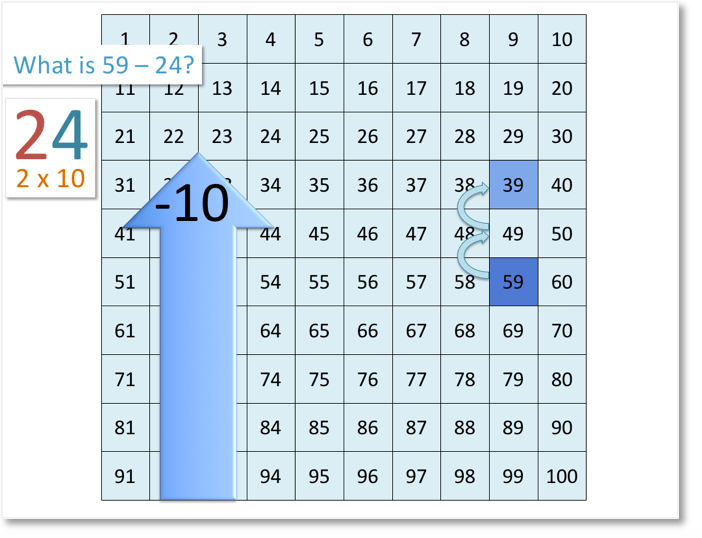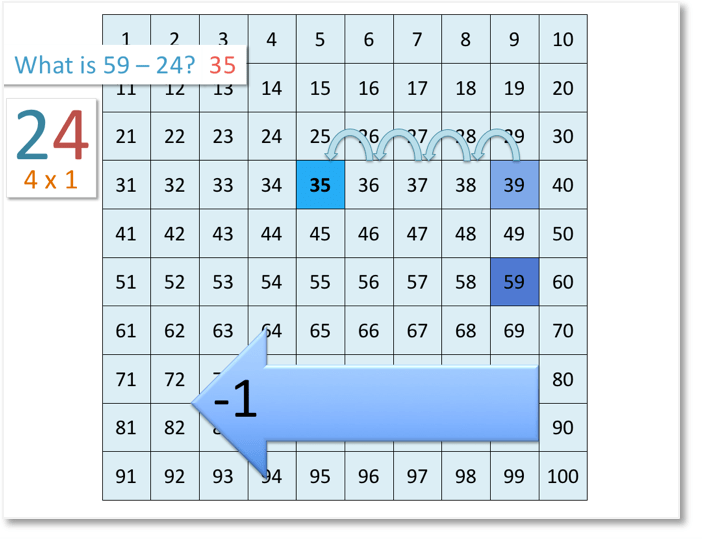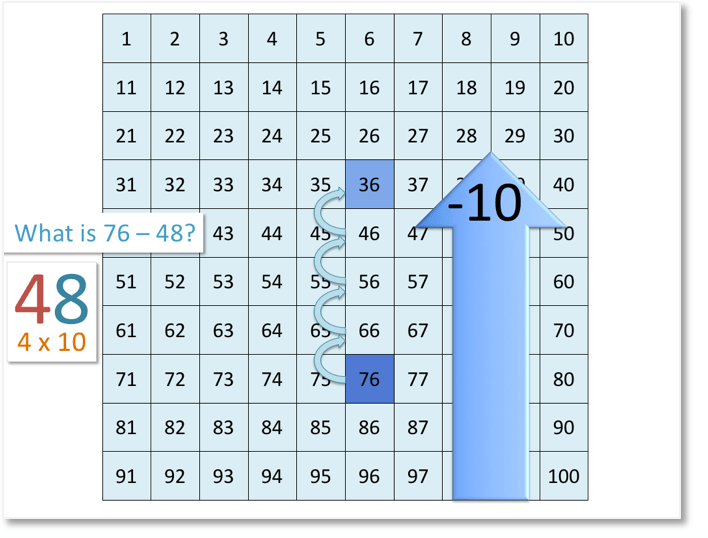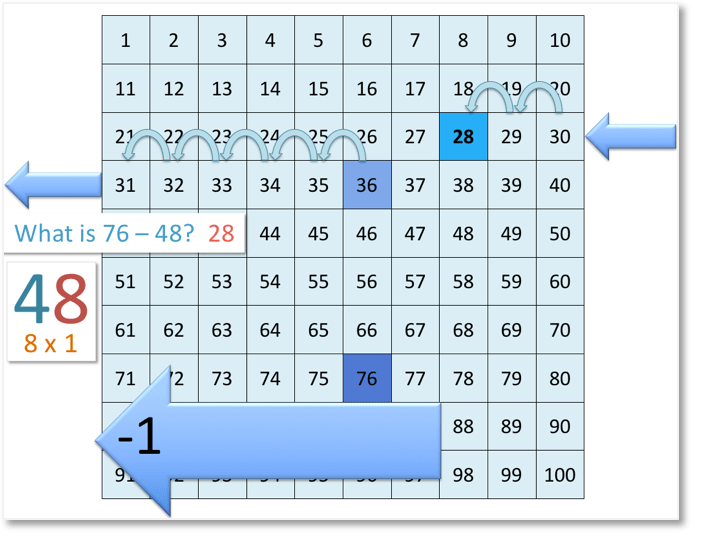# Number Grid: Subtracting Two-Digit Numbers

Number Grid Subtraction: Video Lesson – Maths with Mum Download the Number Grid to 100 below:

Supporting Lessons

## How to Subtract Numbers on the Number Grid

When we subtract tens using the number grid, we are moving up the grid.

When we are subtracting units, we are moving to the left.

In this lesson, we will be looking at subtracting 2-digit numbers using the number grid.

For example:

What is 59 – 24?We’ll begin by looking at the tens. We have 2 in the tens column.

This means that we have 2 lots of 10.

So, we start at 59 and move 2 places up the number grid.

We stop at 39.Next, we’ll look at the units.

We have 4 in the units column. This means that we have 4 lots of 1.

So, we move 4 places to the left on the number grid.

We stop at 35.

Therefore,

59 – 24 =35.

Here is another example:

What is 76 – 48?We’ll begin by looking at the tens.

We have 4 in the tens column. This means that we have 4 lots of 10.

So, we start at 76 and move 4 places up the number grid.

We stop at 36.Next, we’ll look at the units.

We have 8 in the units column. This means that we have 8 lots of 1.

So, we want to move 8 places to the left on the number grid.

After moving 5 places to the left, we stop at 31, which is at the beginning of the row.

We can’t move any more places to the left.

So, we move to the end of the previous row.

So far, we have moved 6 places. We need to move 2 more places to the left. We stop at 28.

Therefore,

76 – 48 = 28.